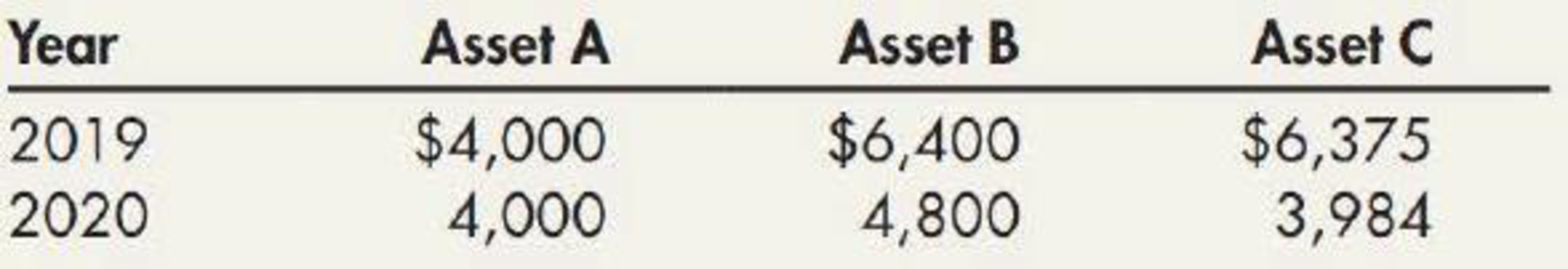Chapter 11, Problem 3E### Intermediate Accounting: Reporting...

3rd Edition
James M. Wahlen + 2 others
ISBN: 9781337788281

#### Solutions

Chapter
Section### Intermediate Accounting: Reporting...

3rd Edition
James M. Wahlen + 2 others
ISBN: 9781337788281
Textbook Problem
85 views

# Depreciation Methods Nickle Company purchased three identical assets for $17,000 on January 2, 2019. Each asset has an expected residual value of$1,000. The depreciation expense for 2019 and 2020 is shown below for three assets:Required: 1. Next Level Which depreciation method is the company using for each asset? 2. Compute the depreciation expense for 2021 and 2022 for each asset.

1.

To determine

Identify the deprecation method that the company has used for each asset.

Explanation

Depreciation: Depreciation is a method of reducing the capitalized cost of long-lived operating assets or plant assets for the period the asset is used.

Straight-line depreciation method: The depreciation method which assumes that the consumption of economic benefits of long-term asset could be distributed equally throughout the useful life of the asset is referred to as straight-line method.

Sum-of- the-years’ digits method:  Sum-of-the years’ digits method determines the depreciation by multiplying the depreciable base and declining fraction.

Double-declining-balance method: The depreciation method which assumes that the consumption of economic benefits of long-term asset is high in the early years but gradually declines towards the end of its useful life is referred to as double-declining-balance method.

Identify the deprecation method that the company has used for each asset as follows:

Asset A:

In this case, the company has used straight line method for Asset A, because depreciation expense for each year is same.

Calculate the straight line rate for Asset A.

Depreciation rate = Depreciation expenseDepreciation base=$4,000$16,000(1)×100=25%

The straight line rate is 25%, which indicates that asset A has useful life of 4 years(10025).

Working note (1):

Calculate the deprecation base of asset.

Depreciation base = Cost of assets Estimated residual value=$17,000$1,000=\$16,000

Asset B:

In this case, the company has used sum of the year’s digit method for Asset B, because depreciation expense for each year is decreased by the fraction

2.

To determine

Calculate the depreciation expense of Company N for 2021 and 2022 for each asset.

### Still sussing out bartleby?

Check out a sample textbook solution.

See a sample solution

#### The Solution to Your Study Problems

Bartleby provides explanations to thousands of textbook problems written by our experts, many with advanced degrees!

Get Started